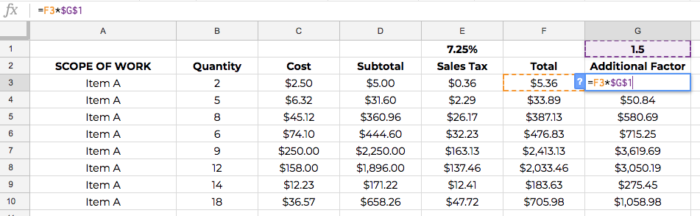# HOW TO ESTIMATE A CONSTRUCTION PROJECT – SPREADSHEET FUNCTIONS## CLICK BELOW TO WATCH A VIDEO TUTORIAL OF THIS POST.

Learning how to estimate a residential construction project takes years of experience both in the field and in the office.

For the construction business owner gaining the experience in the fieldwork is the fun part, and the office work is drudgery.

You produce the fieldwork by building things with your hands.

The office work is hours of staring at a computer screen, often a spreadsheet, trying to produce a proposal for work.

You know how to build it. You think you know how much it should cost, but you are guessing without accurate estimating systems in place.

In this article on FineHomebulding.com, I show you three simple spreadsheet functions that will demonstrate how to estimate a residential construction project in less time and with more accuracy.

## 3 SIMPLE SPREADSHEET FUNCTIONS FOR ESTIMATING CONSTRUCTION PROJECTS

The 3 simple spreadsheets functions* are:

1. COPY and PASTE
2. ABSOLUTE REFERENCE
3. DATA VALIDATION

* The format for the examples and functions in this post are for Google Sheets. To my knowledge, the functions are similar (if not the same) to functions used with .xls files.  Also, I use a Mac. Where I refer to keyboard strokes, I am referring to the Mac OS keyboard strokes. You may need to modify the keystrokes listed here for your particular operating system.

## CONSTRUCTION ESTIMATING SPREADSHEET FUNCTION NUMBER 1 – COPY and PASTE

Copy and Paste is one of the most basic functions for a spreadsheet. This function is very similar to the function for most word processing, except in spreadsheets you can copy and paste formulas as well as text.

For example:

Here’s a basic layout for a proposal.If you wanted to COPY the text ‘Item A’ from cell A3 and populate the cells below A3 with the same text, you can do this in several ways.

COMMAND-C, highlight desired location of pasted cells by clicking and dragging the cursor, COMMAND-VYou can also COPY and PASTE by clicking the small solid box at the lower right-hand corner of the highlighted cell and dragging the mouse to the desired location.

I call this the ‘CLICK and DRAG’ method.

Click the video above to watch a short tutorial on the ‘CLICK and DRAG’ method for COPY and PASTE.

Use the ‘CLICK and DRAG’ method not only for text, but formulas as well.

The Sub-Total in Column D above is calculated by the following formula:

=B3*C3

where B3 is the Quantity and C3 is the Cost per Unit = 2 x \$2.50 = \$5.00The ‘CLICK and DRAG’ method allows you to repeat the calculation for each of the values of Quantity and Cost.

Once the formula is set in cell D3, all you have to do is CLICK and DRAG the selected cell down to row 10. The cells in Column D will now show the result of the formula:

Quantity x Cost = SubtotalThis is the basic function of COPY and PASTE.

Now, let’s calculate the cost of Sales Tax for each line item.

And this is where the next function comes in handy.

## CONSTRUCTION ESTIMATING SPREADSHEET FUNCTION NUMBER 2 – ABSOLUTE REFERENCES

In the COPY and PASTE example above, the Quantity and Cost changed for each item as the formula for the Subtotal was copied.

But in order to calculate the Sales Tax, you only need to multiply one number by the amount calculated for each Subtotal.

The formula for the Sales Tax in \$ = Subtotal x Sales Tax %
=D3*\$E\$1The ‘\$’ on either side of the ‘E’ in the formula above make cell E1 (the location of the value of the Sales Tax%) an Absolute Reference.

You can use an absolute reference to keep a row and/or column constant in any formula.

Since the Sales Tax of 7.25% is fixed in place, we can use the ‘CLICK and DRAG’ method to populate the value of the Sales Tax for each line item.We can repeat the ‘CLICK and DRAG’ method to calculate the Total for each line item:

Total = Subtotal + Sales Tax

Or

=D3+E3

And we can add an Additional Factor (markup perhaps) to the Total to determine the final value.Again, make cell G1 an absolute reference by adding a ‘\$’ before and after the ‘G’.

Now you can COPY and PASTE the formula in G3 from row 3 to row 10.

Learning how to use the various Copy and Paste methods with cell references will speed up time it takes to calculate the numbers in your proposals.

And once you have a spreadsheet built with the formulas, then you don’t have to start from scratch.

You can copy your proposal file and change the data.

Even though the quantities change from job to job, most residential construction businesses can standardize their scopes of work.

And this is where it third spreadsheet function comes in.

## CONSTRUCTION ESTIMATING SPREADSHEET FUNCTION NUMBER 3 – DATA VALIDATION

Standardizing your scope items can greatly increase the accuracy and speed of preparing proposals.

In this first example, I showed you how to Copy and Paste ‘Item A’ from row 3 to row 10.

But what if you wanted to list a unique scope item for each row?

Use the Data Validation to do this.

See this video on how to set it up in your spreadsheet.

This is what your sheet will look like when you have Data Validation set up:You can use Data Validation to create database of scope items, employees, tools, or anything else you want in list form.

Data Validation can be used in many ways and combined with many other formulas to automate your estimating system.

Click here to see a video showing each of these functions used in the sample spreadsheet above.

Residential construction projects vary in size and scope, but your system for estimating them should not.

Your estimating system should be repeatable, scalable, and quick.